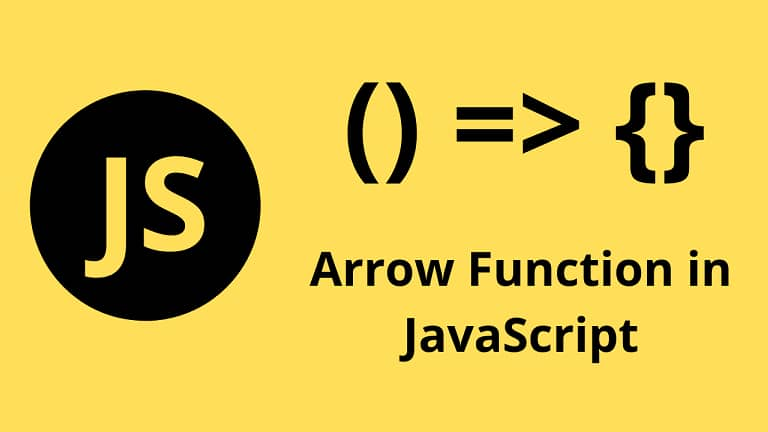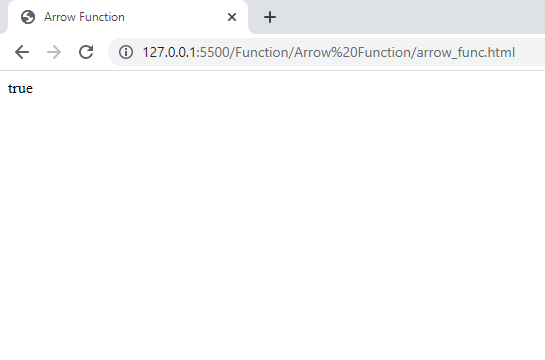# Arrow Function In JavaScript

in STEMGeeks2 years agoImage Source

Introduction of arrow function is another major feature introduced in JavaScript ES6. The name comes from the symbol of arrow (=>) that it utilizes. Arrow functions work similar to like that of anonymous function in Python programming since you don't have to write the function name as well as you don't have to use the keyword function. The main advantage of using arrow function is that it offers a clear and concise way of writing a function. Consider a simple function declaration for adding two numbers:

``````let findSum = function(a, b)
{
return (a+b);
}
``````

In JavaScript we can assign a function to variable as well since functions are also considered as objects in JavaScript. Using arrow function, you can write above function as:

`let findSum = (a, b) => x + y;`

If there is a single parameter, let us consider we are finding square of a number then the arrow function for such scenario would be:
let findSquare = a => a*a;

If there are no parameter required for a function then we write empty parenthesis like: `let greetHello = () => alert("Hello!");`

Whatever the statements are written after the arrow, it is going to be returned, so the keyword return is also not used here . And to call the function you can use the variable name. Lets see a simple program that checks if the given number is even and returns true if it is even and false if it is not even.

``````<!DOCTYPE html>
<html lang="en">
<title>Arrow Function</title>
<script>
let is_even = a =>  a%2==0;

document.write(is_even(6));
</script>